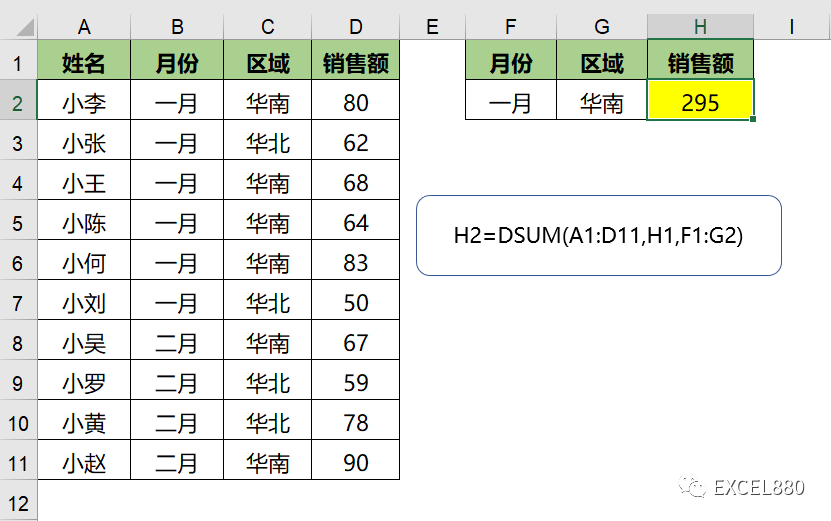# 掌握6种Excel条件求和技巧 – 求和策略完全指南

156 次浏览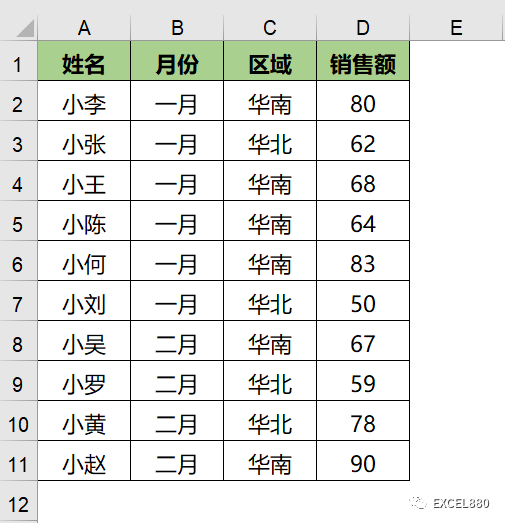1 SUMIF

SUMIF只能单条件求和。如下，求取“华南”区的销售额。

=SUMIF(C:C,F2,D:D)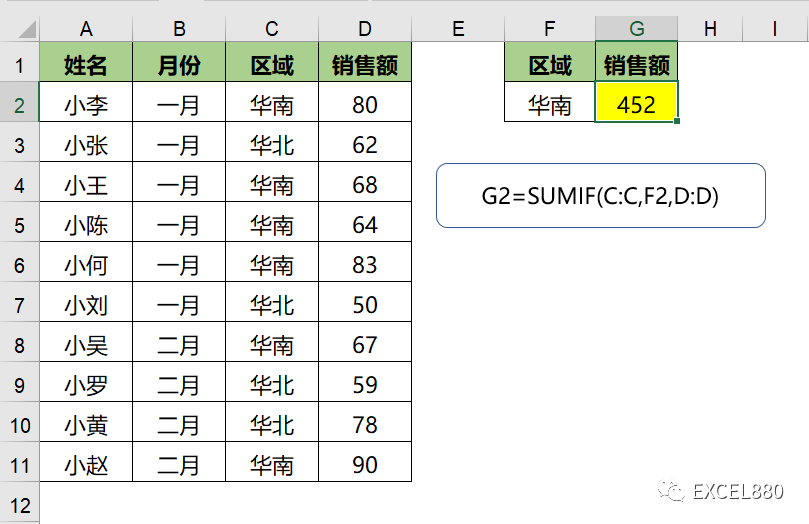2 多条件求和SUMIFS

=SUMIFS(D:D,B:B,F2,C:C,G2)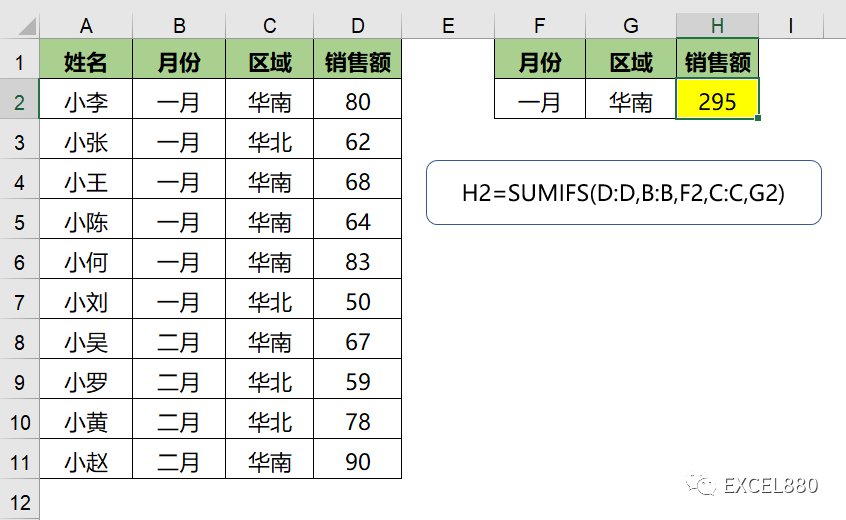3 SUM+IF条件求和

IF函数的数组应用将返回满足条件的所有值，再用SUM求和。逻辑上与SUMIF/SUMIFS雷同。

=SUM(IF(C:C=F2,D:D))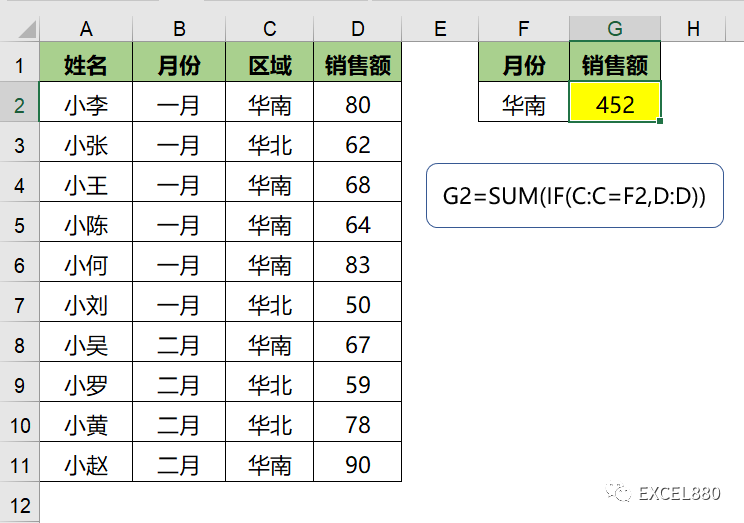4 SUM数组

=SUM((B2:B11=F2)*(C2:C11=G2)*(D2:D11))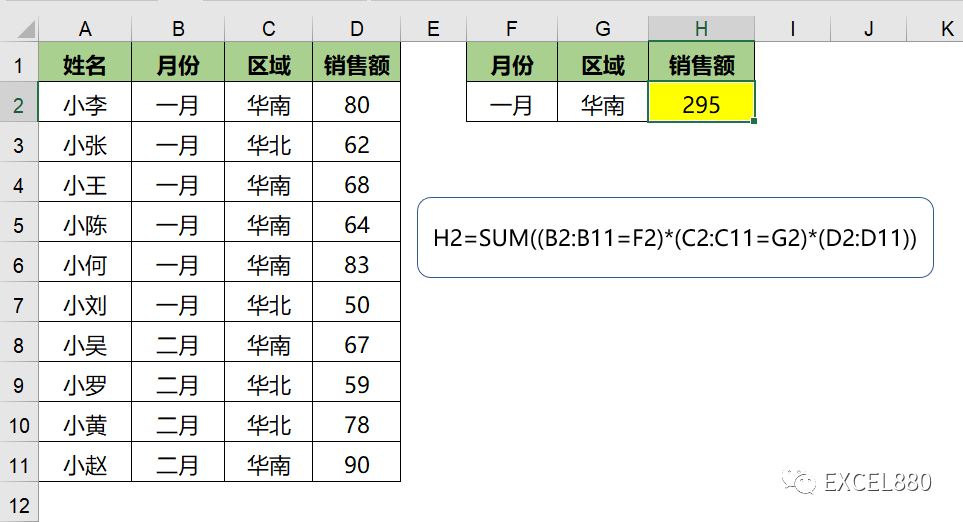5 FILTER筛选求和

Filter的作用是按条件筛选数据，筛选后求和即可。

=SUM(FILTER(D2:D11,(B2:B11=F2)*(C2:C11=G2)))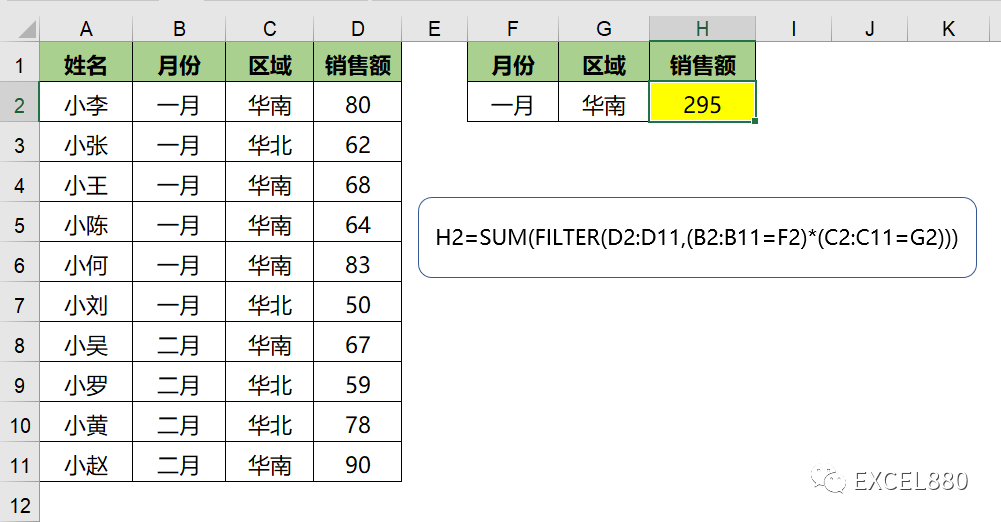6 DSUM数据库求和

=DSUM(数据区域,求和数据区域标题,条件区域)

DSUM是数据库函数，用于返回满足数据库的某列中，满足指定条件的数字之和。

=DSUM(A1:D11,H1,F1:G2)